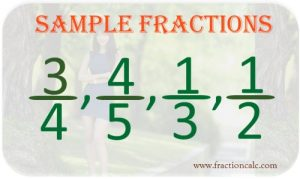## What is Fraction?

Fraction is used by us day by day. We may not aware of it but it is there. When we say, “one piece of pie”, “a quarter of lemon”, “one half of population”, and “two-thirds of flocks”, we are using fractions. Fraction has become part of us. It is impossible to do our daily chores without touching fractions. Even in our jobs, we are using fractions. The ancient people are using fractions. In wars and in peace, fraction is used. Fractions exist as long as human exist. But it was not studied in the earlier days. But when people become sophisticated, when education has been developed, fraction has been studied and even one of the reasons for technological advancement.

The concept of fraction was born when there are too many people for something or when something is not enough for everybody. Even a family of five, when there is only a cake on the table; fraction will be used to divide the cake for the five of them. If there is only one apple for three people, it cannot be divided without using fraction. It should be divided and each of them would receive 3 parts of apple. Even the spoils of wars will be divided through fractions when there are too many soldiers to take. Roman soldiers divided the land they conquered using fractions.

Fractions are just parts of the whole. Anything which is less than a whole is a fraction. It shows how many parts the whole has been divided into and how many parts to count. It shows how many parts needed to make up the whole things.

Fraction is represented in the form of two numbers separated by line. ½ is a fraction representing a whole number divided by two parts. The upper number is called numerator and the bottom number is called denominator. The line is what separates them. The numerator is the number representing the parts that are currently dealt with or the parts that has been used or about to be consumed. The denominator represents how many parts the whole has been divided into. If the apple has been divided into three equal parts, the denominator is three.

See photo below for the example of fractions.Since fraction has been used daily and is part of our lives, rules have been established to deal with the math operations needed for fraction. There are rules on how to add and subtract fractions and rules on how to multiply and divide fractions.

Over the years, the usage and terms of fractions has evolved. There are now types of fractions; in fact, there are three types of fractions namely: proper fraction, improper fraction, and mixed numbers. What we have been talking about above is a proper fraction and this fraction has always a numerator less than the denominator. When numerator is equal or bigger than the denominator, it is called improper fraction. Improper fractions are used when there is a need to use math operation such as multiplication and division. Mixed numbers are whole numbers with fraction such as 1½ and 2¾. When multiply or dividing mixed number, we need to convert it first to improper fraction before we can proceed the operation to get the correct results. The results would be an improper fraction and we need it to convert to mixed number for proper representation.

Copyright © 2019. www.fractioncalc.com                    Privacy Policy | DMCA Policy | Terms Of Service | Contact Us